Home >> Excel Tutorials from Compute Expert >> Excel Tips and Trick >> How to Add Leading Zeroes in Excel

In this tutorial, you will learn how to add leading zeroes in excel using various methods.

When inputting numeric data in excel, we may sometimes need to input a number with leading zeroes. This is probably the case when we need to input something like telephone numbers or ID numbers.

If we just input the number with leading zeroes normally, then excel will remove those leading zeroes automatically. That is because excel immediately converts any number we input into its number form. As we usually write a number without zeroes in front of it, excel applies that assumption to our number input too.

So, what we can do if we should input numbers with leading zeroes in them? We will introduce seven methods for you to be able to do that in excel in this tutorial!

Therefore, read this tutorial until its last part. After that, you can pick the method you prefer to implement to add the leading zeroes to your number.

Disclaimer: This post may contain affiliate links from which we earn commission from qualifying purchases/actions at no additional cost for you. Learn more

## How to Add Leading Zeroes in Excel 1: Using a Single Quote Sign (‘)

The first method to add leading zeroes in excel that we will discuss here is by using a single quote sign. Just type a single quote before you type your number with the leading zeroes and excel won’t remove those zeroes!

Why does that work? Well, by adding the single quote, we tell Excel that the data we type is a text. Thus, Excel won’t automatically convert it into its number form and therefore, our zeroes will still be there!

Need proof? Take a look at the example below.

Let’s say we want to input 0123456789 to a cell in excel. If we type the number directly, then excel will immediately convert it to 123456789 without the 0.However, if we add a single quote before we type 0123456789, the leading zero will still be intact for us!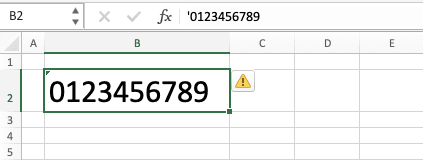A simple method, isn’t it?

## How to Add Leading Zeroes in Excel 2: Using Text Data Format

Another method you can use to add leading zeroes in excel is by changing your cell data format to text.

Just like the single quote method, we use the fact that excel won’t remove leading zeroes from a number text here. However, instead of typing a single quote before the number, we change the cell data format for that.

The way to implement this method is also simple.

Before you type your number with the leading zeroes, highlight the cell where you want to type it. Then, go to the Home tab, click the cell format dropdown button, and choose Text from the dropdown list.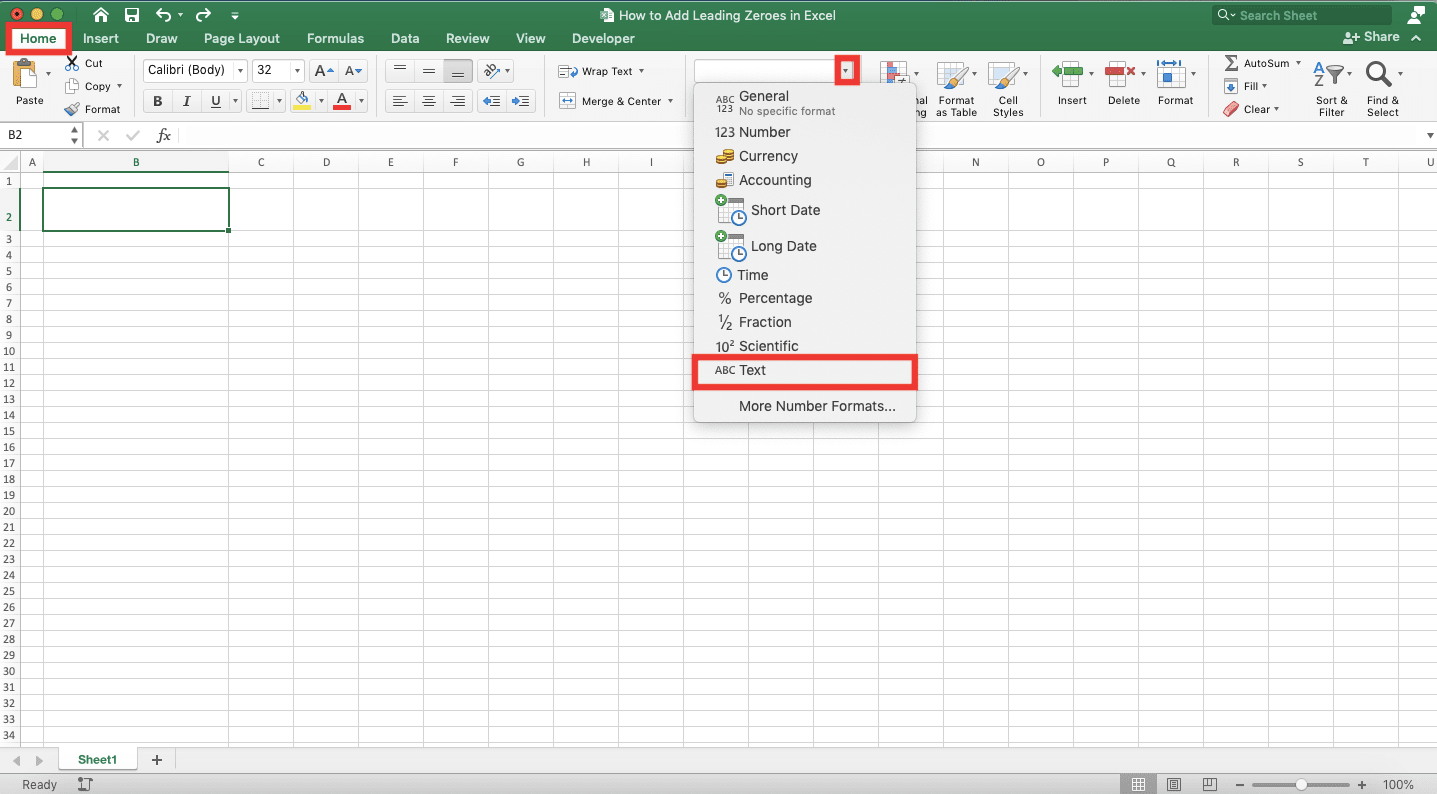After you have done that, just type your number on that cell. Excel won’t remove the leading zeroes in the number after you finish typing it!## How to Add Leading Zeroes in Excel 3: Using Custom Data Format

Besides text data format, you can also use custom data format to add leading zeroes to your number.

You can do this before or after you type your number in a cell. Highlight the cell for the number with leading zeroes and go to the Home tab.

Then, click the cell format dropdown button and choose More Number Formats…. Alternatively, you can just press Ctrl + 1 (Command + 1 in Mac) buttons after you highlight the cell.In the dialog box that shows up, go to the Number tab and click Custom in the left box.Then, just type zeroes in the text box. The number of zeroes you should type equals the sum of the number of leading zeroes and your number length.

For example, for the example number (123456789), the length is 9 (there are 9 numbers there). Just add that to the number of leading zeroes we want to get the number of zeroes we should type.

For this example, let’s say we want 3 leading zeroes. Thus, we type 12 zeroes in the custom data format text box in the dialog box.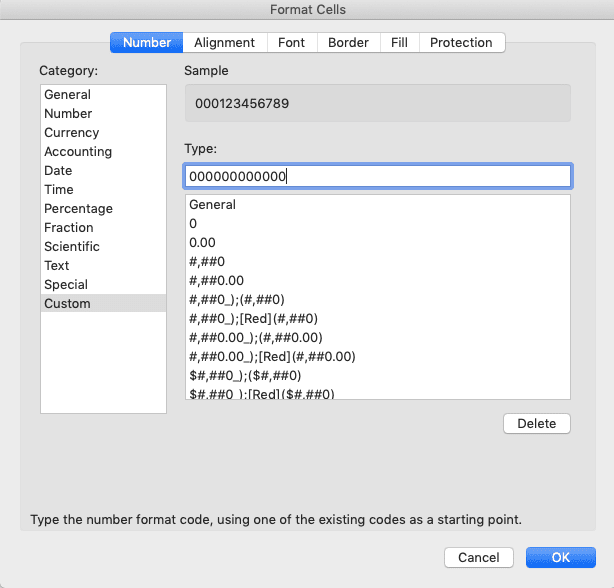You can see the sample of the number display you will get above the text box.

After you finish typing the zeroes, just click the OK button in the dialog box.You will immediately get your number with the leading zeroes you want on display!## How to Add Leading Zeroes in Excel 4: Using TEXT Formula

We can also use excel functions to help us add leading zeroes into our number. The first function we can use for that purpose is TEXT.

TEXT is an excel function that can change the display format of our data. By using it, we can also add leading zeroes to a number.

Here is the general writing form of the TEXT formula for the purpose.

= TEXT ( number , zeroes )

Just input the number we want to add leading zeroes into and the zeroes in quotes.

The principle of the zeroes input is similar to what we have discussed in the custom data format method previously. We must type zeroes as many as the sum of our number length and the number of leading zeroes to add.

Here is the implementation example of the TEXT formula writing in excel.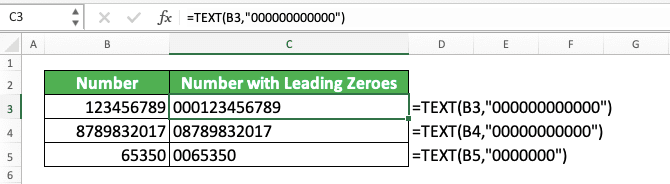The number we input to TEXT will become a text in the TEXT result.

## How to Add Leading Zeroes in Excel 5: Using CONCATENATE Formula

Here is the general writing form of CONCATENATE for this purpose.

= CONCATENATE ( leading_zeroes , number )

CONCATENATE will combine your number with the leading zeroes and excel won’t remove those zeroes from the CONCATENATE result!

Here is the implementation example of the CONCATENATE formula writing in excel.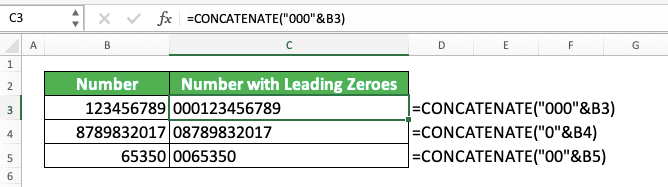Pretty simple, right? Just input the number of leading zeroes you want and your number too to CONCATENATE and press enter. You will immediately get your number with the leading zeroes that way!

## How to Add Leading Zeroes in Excel 6: Using BASE Formula

Another formula you can use to add leading zeroes to your number is BASE. You need to give 10 as the BASE radix input here so it will produce a normal number form. You need to also input the resulting length you want after you add the leading zeroes to your number.

= BASE ( number , 10 , sum_of_number_length_and_number_of_leading_zeroes_we_want )

And here is the implementation example of the BASE formula writing in excel.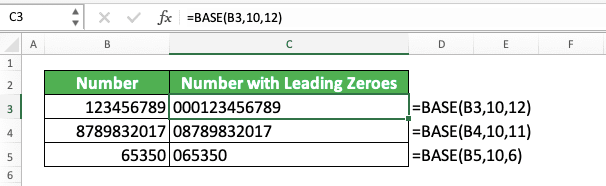BASE will add zeroes in front of your number if you request a number length more than what your number has. As such, we can use it to add leading zeroes to our numbers as you can see in the example.

## How to Add Leading Zeroes in Excel 7: Using the Ampersand Symbol (&)

Using the same principle as CONCATENATE in adding our leading zeroes, you can also use the ampersand symbol for the purpose. Just type the leading zeroes in quotes, the ampersand symbol, and input your number to do this.

Here is the general writing form of the formula.

And here is the implementation example of that in excel.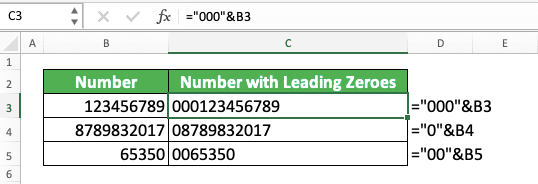## How to Remove Leading Zeroes in Excel

What if you want to do the opposite, which means you want to remove leading zeroes from a number?

There is an excel function you can use for that matter. That function is VALUE.

The way to use VALUE is quite easy too in excel. You just have to input the number with the leading zeroes you want to remove like this.

Press enter after you write that and you will immediately get the number without its leading zeroes.

Here is the implementation example of VALUE for the leading zeroes removal purpose in excel.If the number with leading zeroes is not a result of a formula, you can just change its data format. To do that, highlight that number, go to the Home tab, click the cell format dropdown button, and then choose General.By doing that, you will get the number form without its leading zeroes.

## Exercise

After you have learned how to add (and remove) leading zeroes in excel completely, let’s do this exercise to practice your lessons!

### Instruction:

Give your answer in the appropriate gray-colored cell according to the question number!
1. Add one leading zero to the number on the left by using a single quote sign!
2. Add five leading zeroes to the number on the left by using the excel TEXT function!
3. Add two leading zeroes to the number on the left by using the ampersand symbol!

Besides adding leading zeroes to numbers, you may also want to add them to other data besides numbers. For that, you should use the formulas and the ampersand symbol methods.

Related tutorials you should learn too:

Get updated excel info from Compute Expert by registering your email. It's free!